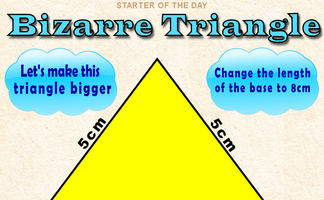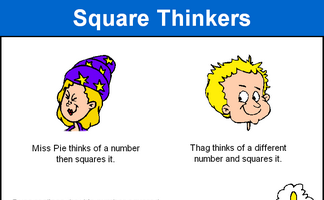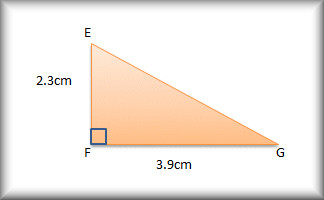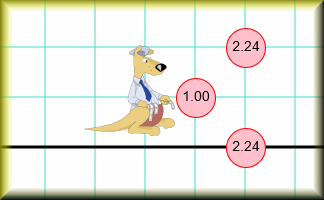#There are 366 different Starters of The Day, many to choose from. You will find below some starters on the topic of Pythagoras. A lesson starter does not have to be on the same topic as the main part of the lesson or the topic of the previous lesson. It is often very useful to revise or explore other concepts by using a starter based on a totally different area of Mathematics.

Main Page

### Pythagoras Starters:By how much would the area of this triangle increase if its base was enlarged to 8cm?Either estimate the lengths of the red lines or, if you know how, calculate how long they are.Can you find three square numbers where the third is the sum of the first two?

## Exercises#### Pythagoras

An online exercise to test your understanding of and ability to apply Pythagoras' Theorem.#### Pythagoras Basics

A drill and practice self marking exercise on the basics of Pythagoras' theorem.#### Where's Wallaby?

Find the hidden wallaby using the clues revealed at the chosen coordinates.

### Search

The activity you are looking for may have been classified in a different way from the way you were expecting. You can search the whole of Transum Maths by using the box below.

Have today's Starter of the Day as your default homepage. Copy the URL below then select
Tools > Internet Options (Internet Explorer) then paste the URL into the homepage field.

Set as your homepage (if you are using Internet Explorer)

Do you have any comments? It is always useful to receive feedback and helps make this free resource even more useful for those learning Mathematics anywhere in the world. Click here to enter your comments.For All: